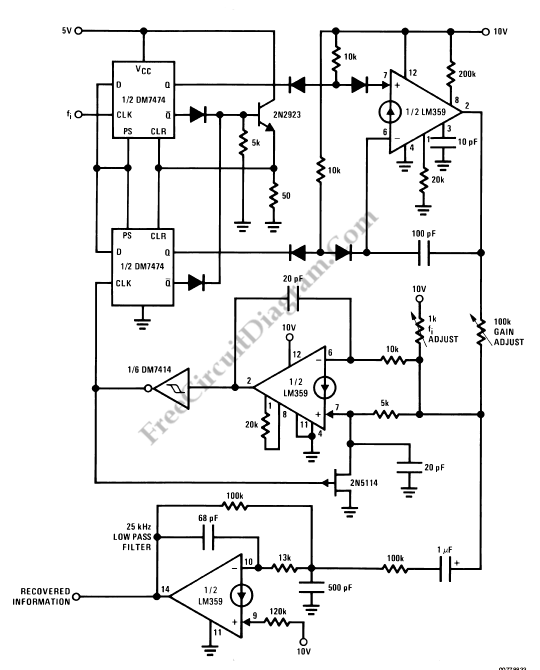## Discrete (Transistors) Schmitt Trigger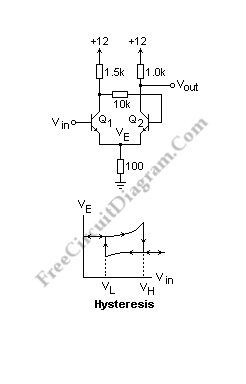Another circuit (from Horowitz and Hill) is shown below. The circuit is almost the same except that the base of Q2 is not connected to the ground. The voltage at the emitters, Ve, is the important thing here. Q1 is off and the output goes low when the base of Q1 rises to 0.7V above Ve if the input is […]

## LM311 Comparator with Hysteresis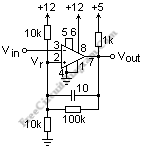An LM311 comparator circuit with the pin numbers  is shown in the schematic diagram figure below. The ground for this transistor is at pin 1 and the open collector output is at pin 7. It is shown pulled up to the logic voltage of +5 with a 1k resistor, which is typical. The LM311 output can sink 8 mA. A […]

## Noninverting Amplifier with Op-Amp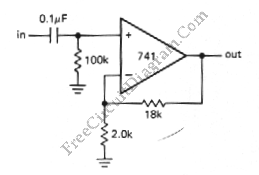This is a noninverting amplifier circuit. This circuit amplify the input voltage with the same phase with output voltage. This circuit has infinite input impedance, but using 741, the input impedance would be hundreds of megaohms. The output impedance is a little, in fraction of an ohm. Here is the schematic diagram of the circuit: This circuit is made for […]

## Inverting Amplifier with Op-Amp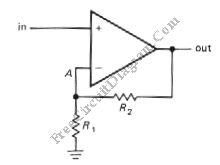This is a Inverting amplifier circuit. This circuit is used to amplify the application that requires large(closed-loop) voltage gain since it has low input impedance. The output of this circuit is  an amplified signal with different phase wit the input. This circuit uses a feedback. Here is the schematic diagram of the circuit: The R1 is 10K and R2 is […]

## Voltage Follower with Op-Amp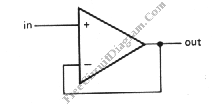This is a emitter follower circuit. This circuit is popular with name buffer circuit. This circuit has gain of 1, so the input voltage is not amplified. Here is the schematic diagram of the circuit: This circuit is a noninverting amplifier with R2 zero and the R1 infinite. This circuit has low output impedance and high input impedance. The LM310 […]

## Op-Amp Voltage Follower With Bootstrap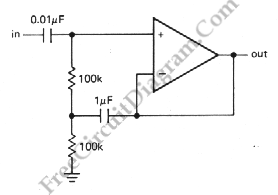The bias path can compromise the high input impedance you would otherwise get with an op-amp as with transistors amplifiers. This particularly with ac-coupled inputs where a resistor to ground is mandatory. The bootstrap circuit shown below is a possible solution if that is a problem. The 1mF capacitor makes the upper 100k resistor look like a high impedance current […]